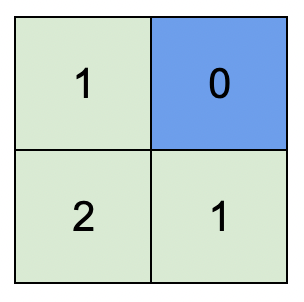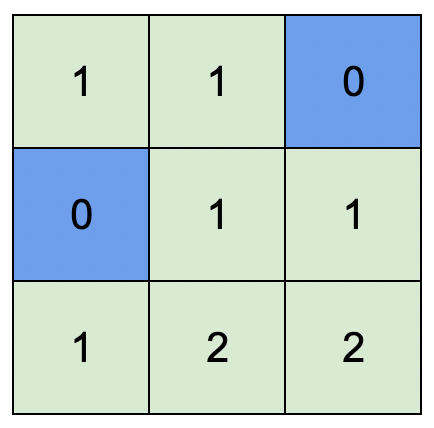Halo

A magic place for coding

0%

Problem

You are given an integer matrix isWater of size m x n that represents a map of land and water cells.

• If isWater[i][j] == 0, cell (i, j) is a land cell.
• If isWater[i][j] == 1, cell (i, j) is a water cell.

You must assign each cell a height in a way that follows these rules:

• The height of each cell must be non-negative.
• If the cell is a water cell, its height must be 0.
• Any two adjacent cells must have an absolute height difference of at most 1. A cell is adjacent to another cell if the former is directly north, east, south, or west of the latter (i.e., their sides are touching).

Find an assignment of heights such that the maximum height in the matrix is maximized.

Return an integer matrix height of size m x n where height[i][j] is cell (i, j)‘s height. If there are multiple solutions, return any of them.

Example 1:Example 2:Constraints:

• m == isWater.length
• n == isWater[i].length
• 1 <= m, n <= 1000
• isWater[i][j] is 0 or 1.
• There is at least one water cell.

Analysis

这道题目给出一个矩阵，里面0表示陆地，1表示水。水的高度一定是0，而相邻两个位置的高度差最多为1，要使得整个矩阵最高的高度是最高的，要求返回最后的矩阵。这一眼就能看出是BFS了，因为相邻的高度差为1，所以只要是相邻我就把高度提高1，这样算出来的高度才是最高的，我们以所有水的位置作为BFS起点，然后维护一个visited矩阵防止重复遍历，最后把整个矩阵返回即可。

无。

Summary

这道题目其实就是经典的BFS加上高度信息。这道题目的分享到这里，感谢你的支持！

Welcome to my other publishing channels